# MAIN

• Introduction

• Materials and methods

•   Device and material

•   Model development

• Results and Discussion

•   Determination of material and frequency of grain separation for perilla and sesame

•   Analysis of high-speed camera images

•   Comparison between experimental and theoretical grain mean transport velocities

• Conclusions

• Conflict of Interest

## Introduction

The devices used for screening cereals employ three types of sorting methods. Screening is performed using mechanical, aerodynamic, or a combination of mechanical and aerodynamic methods (Jung et al., 1992). Generally, a combine uses a straw walker and a cleaning shoe to separate crops from stems and straw. However, combines supplied to Korea do not have a straw walker, and they screen grains by using a cleaning shoe. The grains on the oscillating body pass over the net or louver of the sieve and drop through the hole for cleaning.

The performance of a thresher depends on the efficiency of the oscillator (Jung et al., 1992). The frequency, amplitude, direction of rotation, projection angle (α), and sieve inclination (β) of the oscillator in Figure 1 are important factors of screening performance in the design of screening devices (Kim et al., 1982).##### Figure 1.

Schematic diagram of sieve motion.

A swinging four-bar link structure is mainly used as a screening device for sorting grains. The motions of the swinging body are linear or elliptical. Iwao and Kawamura (1971) derived mathematical equations of the grain transportation for linear, elliptical (Iwao et al., 1971a), and circular (Iwao et al., 1972b) motion. Kludge et al. (1951) described the flying motion of grain with a throwing index (Kv), which is the ratio of excitation acceleration to gravitational acceleration, both of which are components vertical to the sieve. For linear motion, Kim and Gregory (1991) experimentally calculated the mean transport velocity of grains using the throwing index (Kv) and the maximum horizontal velocity of the oscillator. However, in the case of elliptical motion, it is very difficult to determine these values because the characteristics of elliptical motion are different from those of linear motion and the shapes of elliptical trajectories vary on various positions on the surface of the sieve. Hence, the formula for the grain mean transport velocity for various elliptical trajectories on the surface of a test sieve is required. The objective of this study is to propose a formula for the theoretical grain mean transport velocities of an elliptically moving oscillator by modifying the grain mean transport velocity formula applied to linear motion and to compare the calculated values with the experimental values of grain mean transport velocity.

## Materials and methods

Device and material

Figure 2 shows the schematic drawing of the oscillator used in this study. A louver-type sieve was attached to the oscillator. Before the main experiment, to determine the appropriate frequencies of the oscillator and a kind of material, the starting frequencies of grains, stem, and chaff of sesame and perilla were measured at three positions on the sieve surface (start, middle, and end points). The properties of these materials shown in Table 1 were used in the experiment.

Table 1. Physical properties of chaff, grain, and stems of sesame and perilla

 Variety Type Density (kg/m3) Moisture content (%) Perilla Chaff 122 12.9 Grain 446 8.2 Stem 42 11.5 Sesame Chaff 95.7 11.3 Grain 602 5.7 Stem 36.4 10.9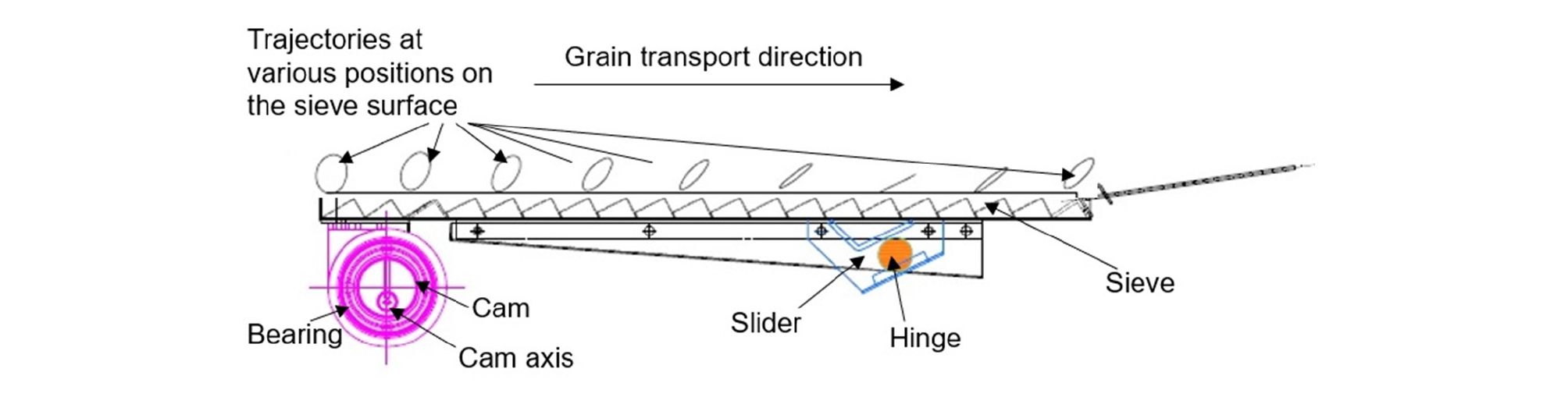##### Figure 2.

Schematic drawing of the oscillator.

As described later in the section of “Results and discussion,” perilla grain was chosen as the material for the experiment. As the oscillating frequencies for the experiment, 200 rpm and 300 rpm were chosen to test the grain mean transport velocity in sliding and flying movements.

In the main experiment, the perilla grains were placed on the plane between the louver scales to prevent small-sized grains from dropping through the louver grooves or being trapped by it. The cam with an eccentric bearing on the oscillator was designed to be eccentric by 1.6 cm so that the lengths of the major axis of the elliptical motion were 3.2–3.6 cm. The camshaft was connected to a 1-HP electric motor by a belt and pulley, and the inverter was connected to adjust the rotating speed. The direction of rotation of the motor was clockwise and the inclination of the sieve (β) was zero. The slider was used as the follower link in the four-bar linkage structure. The slider angle of the slope was 24°.

To compare the theoretical and experimental values of the grain mean transport velocity, the mean transport velocity of the perilla grains was measured using a high-speed camera. The model of the high-speed camera used was NX4-SI/IDT, and the rotation speed of the camshaft was confirmed to be 200 rpm or 300 rpm with a tachometer PLT 200 & ROS-P (Monarch Instrument). The camera resolution was 1000 fps. The velocity of the grains at each position was obtained using an analysis program (Photron FASTCAM Analysis (x64) (Photron Limited)) shown in Figure 3.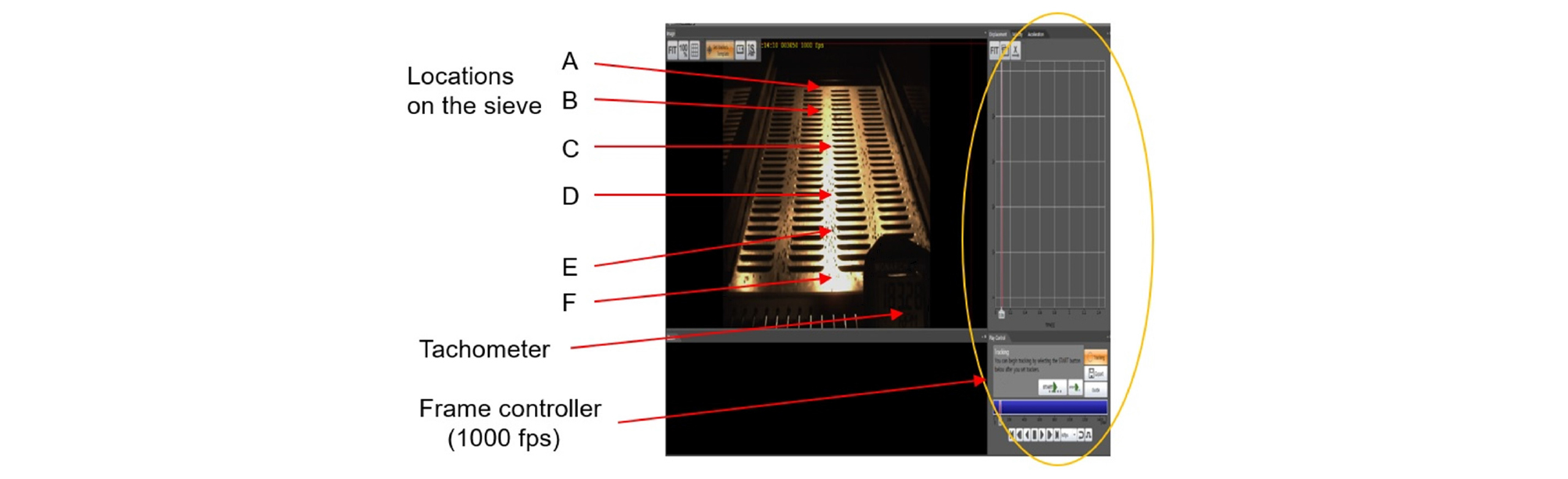##### Figure 3.

Screen display of analysis program (Photron FASTCAM Analysis (x64)).

Model development

The values of Kv and the maximum horizontal velocities for various positions on the elliptical oscillator are obtained using kinematic analysis. To develop the formula for the grain mean transport velocity for elliptical motion, the formula applied to linear motion is modified to consider the angle difference between the maximum accelerations in the directions vertical and horizontal to the sieve. To obtain the actual grain mean transport velocity, the velocities of the perilla grains were measured at six positions on the sieve surface using a high-speed camera and they were compared with the theoretical values.

Throwing index and analysis of linear motion

The throwing index for linear motion is obtained as shown in Figure 4 and equation (1).

For linear motion,

${K}_{V}=\frac{e×{w}^{2}×\mathrm{sin}\left(\alpha +\beta \right)}{g×\mathrm{cos}\beta }=K×\frac{\mathrm{sin}\left(\alpha +\beta \right)}{\mathrm{cos}\beta }$    (1)

where $K=\frac{e×{w}^{2}}{g},$

$e$ : Amplitude of vibration,

$w$ : Rotating speed.##### Figure 4.

Acceleration components in the direction vertical and horizontal to the sieve in the case of linear motion (α: projection angle, β: sieve inclination, a: acceleration of the sieve, an: vertical component of acceleration, a, to the sieve, g: gravitational acceleration, gn: vertical component of acceleration, g, to the sieve).

In the case of linear motion, the initial conditions of forward and backward sliding, and flying are presented in equations (2), (3), and (4), respectively (Jung et al., 1992).

${K}_{1}=\frac{\mathrm{sin}\left(\varnothing +\beta \right)}{\mathrm{cos}\left(\alpha -\beta -\varnothing \right)}$    (2)

${K}_{2}=\frac{\mathrm{sin}\left(\varnothing -\beta \right)}{\mathrm{cos}\left(\alpha -\beta +\varnothing \right)}$    (3)

${K}_{0}=\frac{\mathrm{cos}\beta }{\mathrm{sin}\left(\alpha +\beta \right)}$    (4)

where ∅ = tan-1 µ,

µ : Coefficient of friction.

According to these values and the K value, the forward and backward sliding or flying movements of the grains occur. For example, in the case of K1<K2<K, the sliding of the grain occurs in the forward and backward directions, but it moves in the forward direction rather than the backward direction. If K0<K, the grain flies from the sieve.

For the estimation of the grain mean transport velocity in linear motion, the throwing index (Kv) is used. The mean transport velocity of the grain on the oscillator is given by the following formula (Kim and Gregory, 1991).

Vh = Cs Kv Vhmax    (5)

where Vhmax: Maximum horizontal velocity of the oscillator,

Kv: Throwing index,

Cs: Constant.

In the case of elliptical motion, the values of Kv and Vhmax vary with the positions on the surface of the sieve.

Kinematic analysis of the elliptical motion

As shown in Figure 2, the grains fallen from the threshing section move along the sieve of the oscillator with various elliptical motions from the starting point to the ending point of the sieve. Along the sieve plane, the maximum horizontal velocity and the throwing index (Kv) of the sieve also change. The throwing index is the ratio of excitation acceleration to gravitational acceleration, both of which are components vertical to the sieve.

The major axis (a), minor axis (b) and projection angle (α) of the ellipse, which are shown in Figure 5, also vary according to the positions on the surface of the sieve (Kim, 1982).##### Figure 5.

Elliptical trajectories at various positions on the surface of the sieve.

The velocity and acceleration for each deformed elliptical motion on the sieve are analyzed using the kinematic tool, Working Model 2005. The simulation of four-bar linkage of the oscillator using Working Model 2005 is shown and the trajectories at various positions on the surface of the sieve are illustrated in Figure 6. Working Model 2005 also provides the values of velocities and accelerations at the positions on the surface of the sieve.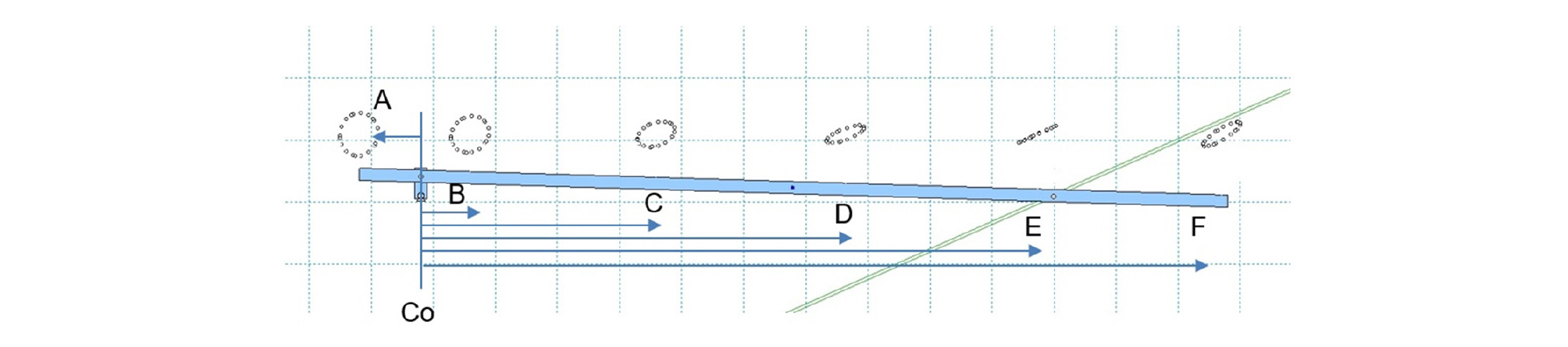##### Figure 6.

Simulated four-bar linkage and trajectories at various positions on the sieve surface.

The six positions on the surface of the sieve were represented by their distances from the cam axis, Co. In Table 2, the amplitudes of the major and minor axes, projection angles, and the distances of the six positions on the surface of the sieve are presented.

Table 2. Major and minor axes and projection angles of ellipses of the six sieve positions

 Positions Major axis: 2a (cm) Minor axis: 2b (cm) Ratio of two axes: b/a Angle of projection: α(degree) Distance of the six positions from Co (cm) A 3.56 3.09 0.87 74 -4 B 3.26 2.72 0.83 29 6 C 3.27 1.77 0.54 21 21 D 3.33 0.79 0.24 20 36 E 3.44 0.29 0.08 25 51 F 3.63 0.97 0.27 33 66

Maximum horizontal velocity and throwing index of the sieve in elliptical motion

Through the kinematic analysis, the maximum horizontal velocities and throwing index (Kv) at the six positions on the sieve surface were obtained (Table 3).

Table 3. Vhmax and Kv values of the six positions obtained using Working Model 2005

 Index and Velocity Frequency Positions Kv Vhmax (cm/s) 200 rpm 300 rpm 200 rpm 300 rpm A 0.79 1.76 31.7 47.6 B 0.67 1.48 31.8 47.7 C 0.47 1.04 31.9 47.9 D 0.31 0.69 32.1 48.1 E 0.31 0.67 32.2 48.3 F 0.46 1.00 32.4 48.5

Theoretical grain mean transport velocity

In the case of linear motion, the maximum accelerations in the directions vertical and horizontal to the sieve are observed at the same angle. Thus, the maximum accelerations in the directions vertical and horizontal to the sieve are determined at the angle where the acceleration in the projection direction becomes the highest.

However, in the case of elliptical motion, the maximum acceleration in the vertical direction to the sieve is not observed at the angle where the maximum acceleration in the horizontal direction to the sieve is observed. Therefore, the influence of the vertical maximum component of the acceleration on the transport velocity of the grain varies according to the angle difference between the maximum accelerations in the horizontal and vertical directions.

The angle difference is defined as the difference between the angle of maximum acceleration horizontal to the sieve and the angle of maximum acceleration vertical to the sieve.

Table 4 shows the maximum accelerations and angle differences at the six positions obtained using the kinematic analysis tool (Working Model 2005) when the rotation speed is 200 rpm.

Table 4. Maximum accelerations in the vertical and horizontal directions of the sieve and angle differences between them

 Positions Maximum acceleration in the vertical direction, cm/s2 (rotating angle, degree) Maximum acceleration in the horizontal direction, cm/s2 (rotating angle, degree) Angle difference between vertical and horizontal maximum accelerations of the sieve (radian) A 775 (180) 690 (264) -1.465 B 652 (180) 685 (264) -1.465 C 460 (192) 676 (264) -1.256 D 308 (216) 667 (264) -0.837 E 299 (276) 658 (264) 0.209 F 448 (300) 649 (264) 0.628

In the case of elliptical motion, the accelerations of the oscillator can be obtained using equations (6) and (7) below (Iwao et al., 1971a).

$\stackrel{¨}{X}=-a{w}^{2}\mathrm{cos}wt×\mathrm{cos}\left(\alpha -\beta \right)-b{w}^{2}\mathrm{sin}wt×\mathrm{sin}\left(\alpha -\beta \right)$    (6)

$\stackrel{¨}{Y}=-a{w}^{2}\mathrm{cos}wt×\mathrm{sin}\left(\alpha -\beta \right)+b{w}^{2}\mathrm{sin}wt×\mathrm{cos}\left(\alpha -\beta \right)$    (7)

The X-axis is the direction along the sieve, and the Y-axis is the vertical direction to the X-axis in Figure 7.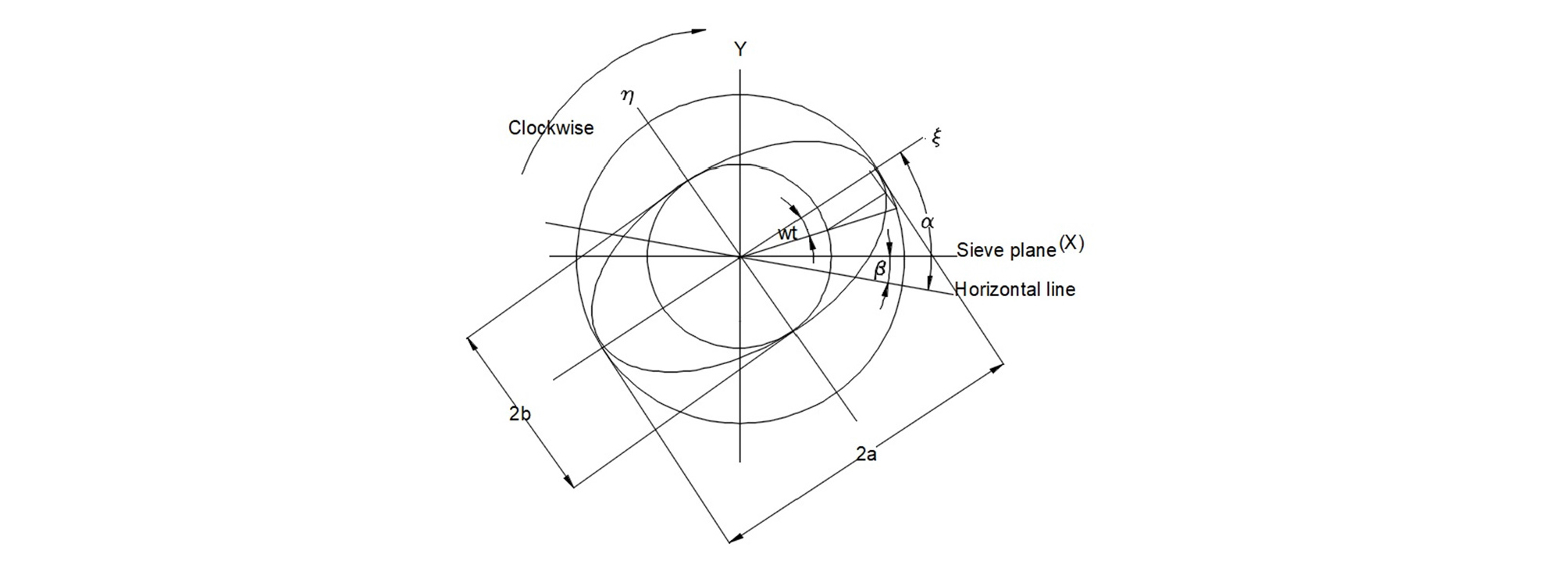##### Figure 7.

Coordinate and variables for the equation of elliptical motion.

The above equations are differentiated to obtain the values of angle (θ=wt) where the maximum accelerations in the directions vertical and horizontal to the sieve are observed, and the angle difference (Δθ) is obtained by equation (8).

$∆\theta ={\mathrm{tan}}^{-1}\left(-\frac{b}{a}\frac{1}{\mathrm{tan}\left(\alpha -\beta \right)}\right)-{\mathrm{tan}}^{-1}\left(\frac{b}{a}\mathrm{tan}\left(\alpha -\beta \right)\right)$    (8)

The computed angle differences calculated using the equation (8) according to the six positions on the sieve surface are as follows (Table 5).

Table 5. Angle difference obtained using theoretical calculation

 A B C D E F Angle difference (radian) -1.497 -1.413 -1.157 -0.670 0.208 l0.567

The values in Table 5 are consistent with the angle differences obtained using the commercial kinematic tool, Working Model 2005, shown in Table 4 (R2 is 0.992).

We can assume that, when the horizontal acceleration is small at the angle of maximum vertical acceleration, the influence of this vertical acceleration on the grain transport velocity is small. In other words, the larger the angle difference between the maximum accelerations in the directions vertical and horizontal to the sieve, the smaller is the influence of the maximum vertical acceleration on the grain mean transport velocity.

The value of Kv derived from the maximum acceleration in the vertical direction to the sieve can be modified using the angle difference (Δθ) when it is used in the formula of the grain mean transport velocity on the elliptical sieve.

Therefore, the formula for the grain mean transport velocity for elliptical motion is expressed in equation (9).

Vh = Cs Kv f(Δθ) Vhmax    (9)

where f(Δθ): Corrective function to adjust the effect of the value of Kv with variable Δθ.

## Results and Discussion

Determination of material and frequency of grain separation for perilla and sesame

As listed in Table 6, the starting frequencies of sliding and flight of the materials differ according to the positions on the sieve surface and the kind of material used.

Table 6. Starting frequencies of sliding and flying of sesame and perilla (rpm)

 Motion Type Positions Materials Sliding Flying Start Mid End Start Mid End Perilla Straw & pod 120 120 120 200 215 230 Grain 180 150 150 240 260 280 Stem 170 200 200 190 230 230 Sesame Straw & pod 115 130 140 140 180 220 Grain 170 160 170 240 260 290 Stem 200 200 230 240 250 240

The perilla grain was chosen as the material for the experiment. The grain moves by sliding on the sieve at a low frequency of the oscillator but it moves with mixed movement of flying and sliding at a high frequency (Iwao et al., 1971). To measure the mean transport velocity of the grain in sliding and mixed movement of flying and sliding, 200 rpm and 300 rpm were chosen as the oscillating frequencies in the experiment.

It has also been considered that it becomes difficult to measure the velocity at each position on the sieve surface if the grains fly too much.

Analysis of high-speed camera images

The perilla grain showed different mean transport velocities according to the positions on the sieve surface and the rotating speeds (Table 7). The grain mean transport velocity increased as the grains moved toward the endpoint.

Table 7. Experimental grain mean transport velocity at the six positions on the sieve surface

 Positions Frequency (rpm) A B C D E F Grain mean transport velocities (cm/s) 200 4.5 3.5 4.0 8.0 9.0 9.0 300 10.0 9.0 10.0 20.0 20.0 20.0

Comparison between experimental and theoretical grain mean transport velocities

With the experimental data of grain mean transport velocity in Table 7, the corrective function, f(Δθ), as an exponential function to represent the S curve (Figure 8) was determined.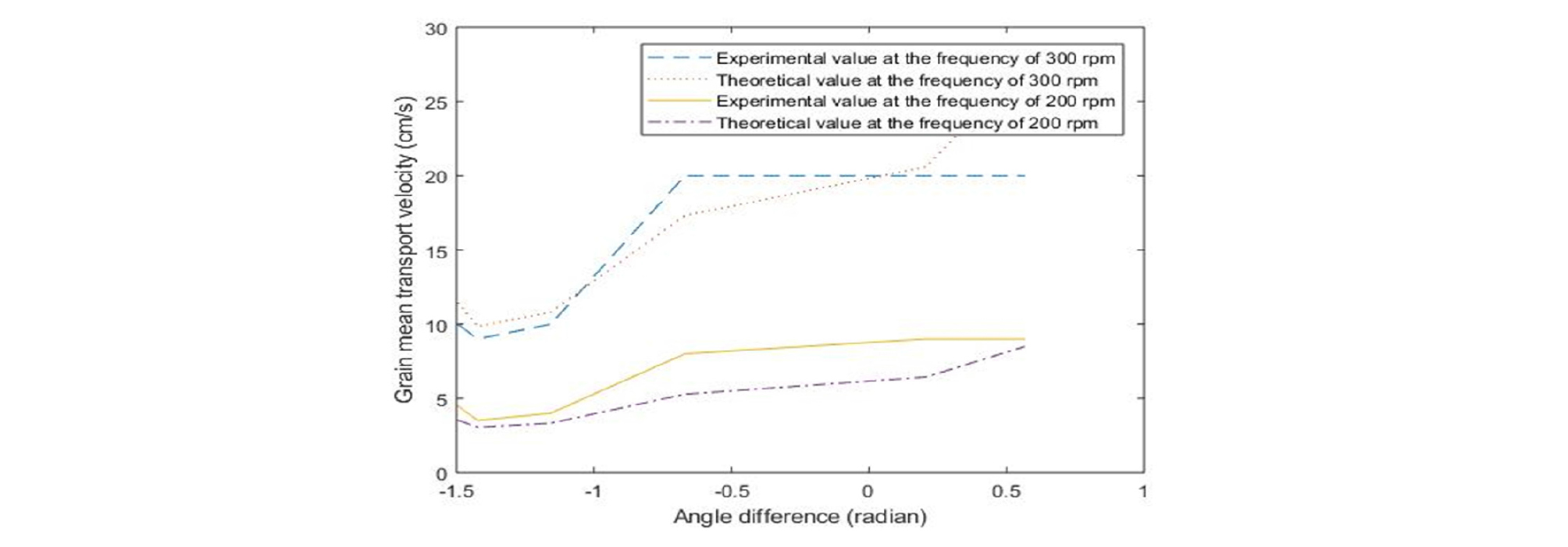##### Figure 8.

Comparison between the experimental and theoretical grain mean transport velocities.

$f\left(∆\theta \right)={C}_{1}\left(1-{e}^{-{C}_{2}\left|∆\theta \right|\left(1-{e}^{-{C}_{3}\left|∆\theta \right|}\right)}+{e}^{{C}_{2}\left|∆\theta \right|\left(1-{e}^{{C}_{3}\left|∆\theta \right|}\right)}\right)$    (10)

where Cs, C1, C2 , and C3: Constants.

Substituting equation (10) into equation (9) yields

(11)

The coefficients of the proposed formula were determined from the experimental data. Cs, C1, C2, and C3 were 0.8, 0.8, 0.16, and 2.0, respectively (R2 is 0.80). Therefore, the equation for the mean transport velocity for the elliptical motion of perilla grains becomes

(12)

In Figure 8, the grain mean transport velocities measured using a high-speed camera are compared with the theoretical values obtained using this equation (RMSE is 2.75).

Thus, it is possible to theoretically obtain the grain mean transport velocities of the screening device in elliptical motion by modifying the formula of the grain mean transport velocities used in linear motion. When this linear formula was applied to elliptical motion without modification (i.e., Vh = Cs Kv Vhmax), R2 for the theoretic and experimental comparison was negative. Hence, the proposed formula might contribute to the analysis of screening with elliptical motion. It is considered that the angle difference between the maximum accelerations in the vertical and horizontal directions to the sieve has as much influence on the grain mean transport velocity as the value of Kv itself. Thus, a kinematic analysis, especially for the accelerations of the sieve, the values of projection angle, and b/a ratio is important for designing a screening mechanism. This study was conducted to investigate the transport velocities of perilla grain only, but further studies on sesame grain, stems, and straw are necessary.

## Conclusions

In this study, the transport velocity of perilla grain on an elliptically oscillating sieve was investigated. It was possible to theoretically obtain the grain mean transport velocities through a screening device with elliptical motion by modifying the formula of the grain mean transport velocity used for linear motion. The formula of the grain mean transport velocity for various elliptical trajectories on the surface of a test sieve was developed. Accordingly, the value of Kv derived from the maximum acceleration in the vertical direction to the sieve could be modified using the angle difference between the maximum accelerations in the directions vertical and horizontal to the sieve. The experimental result was consistent with the theoretical value calculated using the proposed formula. However, the influence of sieve inclination, β, and the properties of the materials should be added for the application of the developed model.

## Conflict of Interest

The authors have no conflicting financial or other interests.

## Acknowledgements

This study was carried out with the support of Rural Development of Administration (RDA-PJ012849032018) and 2018 BK 21 plus (No. 31Z20130013003).

## References

1

Iwao, T. and N. Kawamura. 1971. Motion of grain on the screen surface under reciprocating motion (I). Agricultural Machine Magazine 33(1): 45-52.https://doi.org/10.11357/jsam1937.33.45

2

Iwao, T., N. Kawamura and H. Tanabe. 1971a. Motion of grain on the screen surface under elliptical motion (II). Agricultural Machine Magazine 33(2): 163-172.https://doi.org/10.11357/jsam1937.33.163

3

Iwao, T., N. Kawamura and H. Tanabe. 1971b. Motion of grain on the screen surface under circular motion (III). Agricultural Machine Magazine 33(3): 274-278.https://doi.org/10.11357/jsam1937.33.274

4

Jung, C. J. et al. 1992. Analysis and design of agricultural machinery: Seoul National University Press: 195.

5

Kim, S. H. 1982. Study on separating performance of oscillating sieve in wet-paddy threshing. JKSAM 7(1).

6

Kim, S. H., S. I. Nam and S. N. Ryu. 1984. Study on the characteristics and separating performance of oscillating sieve for optimization of separating losses of combine, JKSAM 9(2).

7

Kim, S. H., and J. M. Gregory. 1991. Grain separation equations for combine chaffers and sieves. ASAE 911603.

8

Kluge, W. 1951. Neuzeitliche Siebmaschinen für die Aufbereitung. Erdöl und Kohle 4. Jahrgang (11): 705-711.

9

Working Model. 2005. Guide works. Version 8.0.1.0. Canton. MI. : Design Simulation Technologies, Inc.